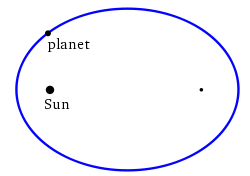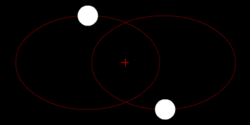# Elliptic orbit

﻿
Elliptic orbitA small body in space orbits a large one (like a planet around the sun) along an elliptical path, with the large body being located at one of the ellipse foci.Two bodies with similar mass orbiting around a common barycenter with elliptic orbits.

In astrodynamics or celestial mechanics an elliptic orbit is a Kepler orbit with the eccentricity less than 1; this includes the special case of a circular orbit, with eccentricity equal to zero. In a stricter sense, it is a Kepler orbit with the eccentricity greater than 0 and less than 1 (thus excluding the circular orbit). In a wider sense it is a Kepler orbit with negative energy. This includes the radial elliptic orbit, with eccentricity equal to 1.

In a gravitational two-body problem with negative energy both bodies follow similar elliptic orbits with the same orbital period around their common barycenter. Also the relative position of one body with respect to the other follows an elliptic orbit.

Examples of elliptic orbits include: Hohmann transfer orbit, Molniya orbit and tundra orbit.

## Velocity

Under standard assumptions the orbital speed ($v\,$) of a body traveling along elliptic orbit can be computed from the Vis-viva equation as:$v=\sqrt{\mu\left({2\over{r}}-{1\over{a}}\right)}$

where:

•$\mu\,$ is the standard gravitational parameter,
•$r\,$ is the distance between the orbiting bodies.
•$a\,\!$ is the length of the semi-major axis.

The velocity equation for a hyperbolic trajectory has either +${1\over{a}}$, or it is the same with the convention that in that case a is negative.

## Orbital period

Under standard assumptions the orbital period ($P\,\!$) of a body traveling along an elliptic orbit can be computed as:$P=2\pi\sqrt{a^3\over{\mu}}$

where:

•$\mu\,$ is standard gravitational parameter,
•$a\,\!$ is length of semi-major axis.

Conclusions:

• The orbital period is equal to that for a circular orbit with the orbit radius equal to the semi-major axis ($a\,\!$),
• For a given semi-major axis the orbital period does not depend on the eccentricity (See also: Kepler's third law).

## Energy

Under standard assumptions, specific orbital energy ($\epsilon\,$) of elliptic orbit is negative and the orbital energy conservation equation (the Vis-viva equation) for this orbit can take the form:${v^2\over{2}}-{\mu\over{r}}=-{\mu\over{2a}}=\epsilon<0$

where:

•$v\,$ is orbital speed of orbiting body,
•$r\,$ is distance of orbiting body from central body,
•$a\,$ is length of semi-major axis,
•$\mu\,$ is standard gravitational parameter.

Conclusions:

• For a given semi-major axis the specific orbital energy is independent of the eccentricity.

Using the virial theorem we find:

• the time-average of the specific potential energy is equal to 2ε
• the time-average of r−1 is a−1
• the time-average of the specific kinetic energy is equal to –ε

## Flight path angle

The flight path angle is the angle between the orbiting body's velocity vector (= the vector tangent to the instantaneous orbit) and the local horizontal. Under standard assumptions the flight path angle ϕ satisfies the equation:$h\, = r\, v\, \cos \phi$

where:

•$h\,$ is the specific relative angular momentum of the orbit,
•$v\,$ is orbital speed of orbiting body,
•$r\,$ is radial distance of orbiting body from central body,
•$\phi \,$ is the flight path angle

## Equation of motion

See orbit equation

## Orbital parameters

The state of an orbiting body at any given time is defined by the orbiting body's position and velocity with respect to the central body, which can be represented by the three-dimensional Cartesian coordinates (position of the orbiting body represented by x, y, and z) and the similar Cartesian components of the orbiting body's velocity. This set of six variables, together with time, are called the orbital state vectors. Given the masses of the two bodies they determine the full orbit. The two most general cases with these 6 degrees of freedom are the elliptic and the hyperbolic orbit. Special cases with less degrees of freedom are the circular and parabolic orbit.

Because at least six variables are absolutely required to completely represent an elliptic orbit with this set of parameters, then six variables are required to represent an orbit with any set of parameters. Another set of six parameters that are commonly used are the orbital elements.

## Solar system

In the solar system, planets, asteroids, most comets and some pieces of space debris have approximately elliptical orbits around the Sun. Strictly speaking, both bodies revolve around the same focus of the ellipse, the one closer to the more massive body, but when one body is significantly more massive, as the sun vis-a-vis the earth, the focus may be contained within the larger massing body, and thus the smaller is said to revolve around it. The following chart of the perihelion and aphelion of the planets, dwarf planets and Halley's Comet demonstrates the variation of the eccentricity of their elliptical orbits. For similar distances from the sun, wider bars denote greater eccentricity. Note the almost-zero eccentricity of Earth and Venus compared to the enormous eccentricity of Halley's Comet and Eris.A radial trajectory can be a double line segment, which is a degenerate ellipse with semi-minor axis = 0 and eccentricity = 1. Although the eccentricity is 1 this is not a parabolic orbit. Most properties and formulas of elliptic orbits apply. However, the orbit cannot be closed. It is an open orbit corresponding to the part of the degenerate ellipse from the moment the bodies touch each other and move away from each other until they touch each other again. In the case of point masses one full orbit is possible, starting and ending with a singularity. The velocities at the start and end are infinite in opposite directions, and the potential energy is equal to minus infinity.

The radial elliptic trajectory is the solution of a two-body problem with at some instant zero speed, as in the case of dropping an object (neglecting air resistance).

## History

Babylonian astronomers in the first millennium B.C.E. observed that the Sun's motion along the ecliptic was not uniform, though they were unaware of why this was; it is today known that this is due to the Earth moving in an elliptic orbit around the Sun, with the Earth moving faster when it is nearer to the Sun at perihelion and moving slower when it is farther away at aphelion.

In the 17th century, Johannes Kepler discovered that the orbits along which the planets travel around the Sun are ellipses with the Sun at one focus, in his first law of planetary motion. Later, Isaac Newton explained this as a corollary of his law of universal gravitation.

Wikimedia Foundation. 2010.

### Look at other dictionaries:

• elliptic orbit — elipsinė orbita statusas T sritis fizika atitikmenys: angl. elliptic orbit; elliptical orbit vok. Ellipsenbahn, f rus. эллиптическая орбита, f pranc. orbite elliptique, f …   Fizikos terminų žodynas

• Orbit equation — In astrodynamics an orbit equation defines the path of orbiting body around central body relative to , without specifying position as a function of time. Under standard assumptions, a body moving under the influence of a force, directed to a… …   Wikipedia

• Orbit — This article is about orbits in celestial mechanics, due to gravity. For other uses, see Orbit (disambiguation). A satellite orbiting the Earth has a tangential velocity and an inward acceleration …   Wikipedia

• Orbit of the Moon — Not to be confused with Lunar orbit in the sense of a selenocentric orbit, that is, an orbit around the Moon The Moon completes its orbit around the Earth in approximately 27.3 days (a sidereal month). The Earth and Moon orbit about their… …   Wikipedia

• Orbit phasing — In astrodynamics orbit phasing is the adjustment of the time position of spacecraft along its orbit, usually described as adjusting the orbiting spacecraft s true anomaly. This is predominantly used in satellite positioning, especially if the… …   Wikipedia

• Bi-elliptic transfer — In astronautics and aerospace engineering, the bi elliptic transfer is an orbital maneuver that moves a spacecraft from one orbit to another and may, in certain situations, require less delta v than a Hohmann transfer.The bi elliptic transfer… …   Wikipedia

• Kepler orbit — Gravitational attraction is the force that makes the Solar system stick together with the planets orbiting the Sun and the Moon orbiting the Earth. Isaac Newton formulated the physical law for this gravitational attraction which explained Kepler… …   Wikipedia

• Geocentric orbit — Earth orbit redirects here. For the motion of the Earth around the Sun, see Earth s orbit. Earth orbiter redirects here. For the shuttle simulator, see Earth Orbiter 1. The following words may have more than one definition or other non Earth… …   Wikipedia

• Hohmann transfer orbit — In astronautics and aerospace engineering, the Hohmann transfer orbit is an orbital maneuver using two engine impulses which, under standard assumptions, move a spacecraft between two coplanar circular orbits. This maneuver was named after Walter …   Wikipedia

• Molniya orbit — For other uses, see Molniya (disambiguation). Figure 1: The Molniya orbit. Usually the period from perigee + 2 hours to perigee + 10 hours is used to transmit to the northern hemisphere Molniya orbit is a type of highly elliptical orbit with an… …   Wikipedia